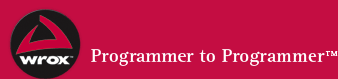Message #1 by "jp inn" on Tue, 12 Jun 2001 17:39:59
```Just use Split.

[example]
Dim arProducts
arProducts =3D Split(Request.Form("myCheckBox"), "'")

For iCount =3D LBound(arProducts) To UBound(arProducts)
Response.Write arProducts(iCount) & "<BR>"
Next 'iCount
[/example]

=3D=3D=3D=3D=3D=3D=3D=3D=3D=3D=3D=3D=3D=3D=3D=3D=3D=3D=3D=3D=3D=3D=3D=3D
=3D=3D=3D=3D=3D=3D=3D=3D=3D
Kyle M. Burns, MCP
Centra Credit Union
kburns@c...

-----Original Message-----
From: jp inn [mailto:jp_inn@y...]
Sent: Tuesday, June 12, 2001 12:40 PM
To: Code Clinic
Subject: [proasp_codeclinic] array

hi.
in a form i have many checkboxes having the same name.
checkbox value stores product number.
i am submitting the form by method post.
how can i retrieve values in calling asp form.

pls give me some example.

thank you

```